# Using Prime Numbers to Understand Machine Learning Classifiers¶

Every number has a unique decomposition into primes. If we enumerate the primes $p_1, p_2, \ldots$ then we can write any positive number $n$ as:

$$n=\prod_{i}{p_i^{\alpha_i}}$$

where $\alpha_i \geq 0$ is the number of factors of the $i$-th prime. A number $n > 1$ is composite if the sum $\sum_i{\alpha_i} > 1$. This is called the fundamental theorem of arithmetic.

In this article we're going to pretend that we don't know that, represent each number as a vector $(\alpha_1, \ldots, \alpha_k)$, and try to use various classifiers to predict if the number is prime or not. The point is to gain some insight into how the classifiers work, not to accurately predict primes.

As we will see, there are some intuitive explainations of how various classifiers work in this context.

To start we import the usual suspects and generate the data. I've hidden the prime generating functions in another file.

In :
import matplotlib.pyplot as plt
import numpy as np
import pandas as pd
%matplotlib inline

plt.rcParams["figure.figsize"] = (8, 8)

In :
from prime_utils import primes_sieve, primes_up_to
from prime_utils import pfs_to_df, simultaneous_prime_factorizations

In :
def generate_data(n):
"""Use the auxilliary functions to generate a data frame
of prime decompositions of all numbers up to n."""
primes = primes_up_to(n)
pfs = simultaneous_prime_factorizations(n, primes=primes)
df = pfs_to_df(n, pfs, primes)
columns = df.columns[1:-1]
X = df[columns]
y = df["is_prime"]
return X, y


Let's take a look at the data.

In :
n = 100
primes = primes_up_to(n)
print(primes)
pfs = simultaneous_prime_factorizations(n, primes=primes)
df = pfs_to_df(n, pfs, primes)
df.tail()

[2, 3, 5, 7, 11, 13, 17, 19, 23, 29, 31, 37, 41, 43, 47, 53, 59, 61, 67, 71, 73, 79, 83, 89, 97]

Out:
number 2 3 5 7 11 13 17 19 23 ... 59 61 67 71 73 79 83 89 97 is_prime
93 95 0 0 1 0 0 0 0 1 0 ... 0 0 0 0 0 0 0 0 0 0
94 96 5 1 0 0 0 0 0 0 0 ... 0 0 0 0 0 0 0 0 0 0
95 97 0 0 0 0 0 0 0 0 0 ... 0 0 0 0 0 0 0 0 1 1
96 98 1 0 0 2 0 0 0 0 0 ... 0 0 0 0 0 0 0 0 0 0
97 99 0 2 0 0 1 0 0 0 0 ... 0 0 0 0 0 0 0 0 0 0

5 rows × 27 columns

Let's plot the data frame next.

In :
fig = plt.figure(figsize=(20, 10))
plt.pcolor(df[df.columns[1:]], cmap="Blues")
plt.colorbar()
plt.ylabel("Number")
plt.xlabel("Columns")
ticks = df.columns[1:]
plt.xticks([x + 0.5 for x in range(len(ticks)+1)], ticks, rotation=45)
plt.tight_layout()
plt.show()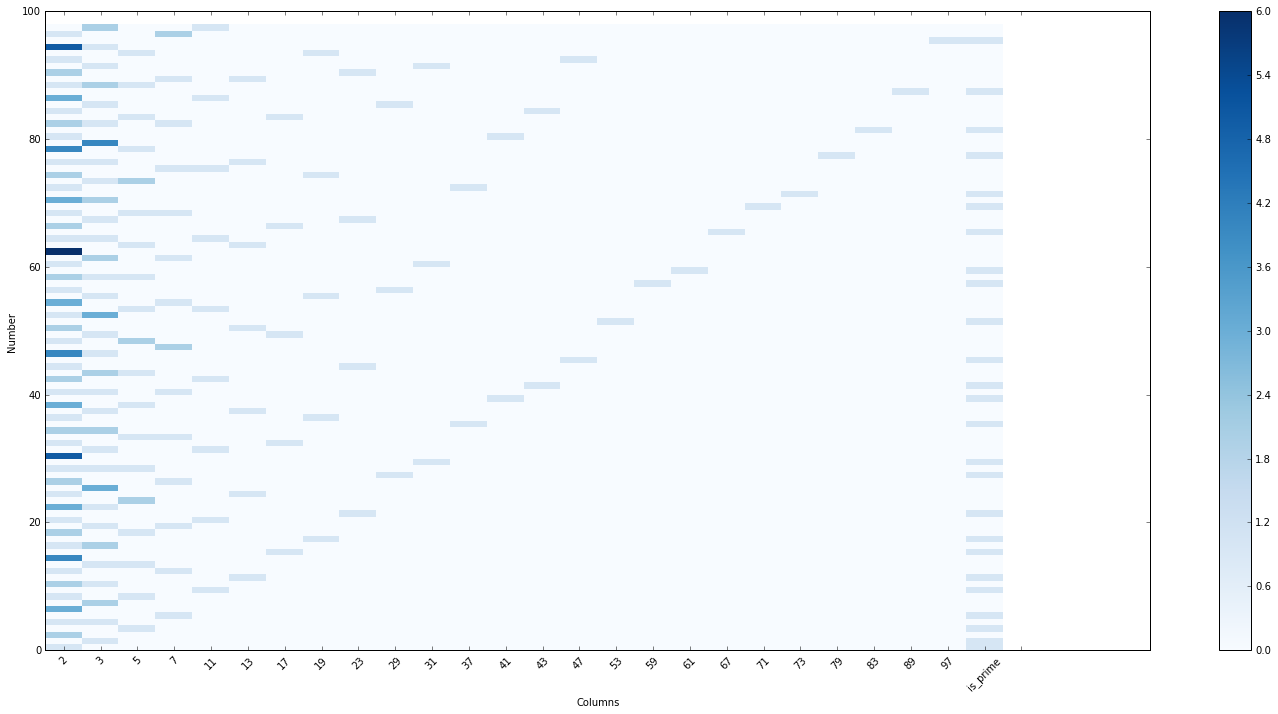## PCA on the Prime Decompositions¶

We can try to reduce the dimensionality of our data with PCA, a matrix transformation technique that finds eigenvectors of the covariance matrix. The resulting vectors correspond to linear transformations of the columns in our data and give the directions which capture the most variance in the data.

In :
from sklearn import decomposition

n = 1000
primes = primes_up_to(n)
pfs = simultaneous_prime_factorizations(n, primes=primes)
df = pfs_to_df(n, pfs, primes)
columns = df.columns[1:-1]

pca = decomposition.PCA(n_components=4)
pca.fit(df[columns])

print("Explained Variances:", pca.explained_variance_ratio_)
print("PCA1:", pca.components_[:5])
print("PCA2:", pca.components_[:5])
print("PCA3:", pca.components_[:5])

Explained Variances: [ 0.46553904  0.17851723  0.07239022  0.04507373]
PCA1: [-0.9998347   0.01212174  0.00687293  0.00329306  0.00232463]
PCA2: [ 0.01179443  0.99959331 -0.013083   -0.01047271 -0.00446868]
PCA3: [-0.0066311  -0.01223476 -0.99919122  0.02081732  0.01058333]


The first component is almost entirely composed of our first column, the number of 2s that divide our numbers. This captures the most variance in whether a number is prime or not, exlcuding half of all numbers, and has an explained variance of almost 0.5.

The second component is mostly composed of the second column, the number of 3s that divide our number. This excludes one third of all numbers, but only one-sixth after we've excluded those divisible by 2. That's why the explained variance is approximately $1/6 = 0.166\ldots \approx 0.1778$.

You probably see the pattern. The third component should explain approximately 1/30th of the data since $30 = 2*3*5$. It's not exactly equal since we start to get more complex cross-correlations as the number of primes grows, and there is some variation in the PCA weightings depending on the convergence limits of the algorithm.

## Classification¶

Let's try to use our data frame to predict if a number is prime or not. Of course we can easily tell from a prime factorization if a number is prime or not, so we're mainly doing this to gain intuition about the classification algorithms. Let's define a few helper functions to plot our results.

In :
from sklearn.metrics import accuracy_score, confusion_matrix

def plot_confusion_matrix(cm, title='Confusion matrix', cmap=plt.cm.Blues):
plt.imshow(cm, interpolation='nearest', cmap=cmap)
plt.title(title)
plt.colorbar()
names = ["Composite", "Prime"]
tick_marks = np.arange(len(names))
plt.xticks(tick_marks, names, rotation=45)
plt.yticks(tick_marks, names)
plt.tight_layout()
plt.ylabel('True label')
plt.xlabel('Predicted label')

def plot_probabilities(X, clf):
"""Plots the predicted probability that a number is prime."""
probabilities = clf.predict_proba(X)
plt.scatter(range(len(probabilities)), [p for p in probabilities])
plt.ylabel("Predicted probability of primality")
plt.xlabel("Integer N")
plt.show()

def fit_and_predict(X, y, clf, plot_prob=True):
clf.fit(X, y)
predictions = clf.predict(X)
acc = accuracy_score(y, predictions)
cm = confusion_matrix(y, predictions)
return acc, cm, clf

def misclassified_values(X, y, clf):
"""Determine which numbers are incorrectly predicted."""
pred = clf.predict(X)
misses = []
for i, (y_pred, y_true) in enumerate(zip(pred, y)):
if y_pred != y_true:
misses.append(i+2)
return misses


### Decision Tree Classifier¶

We can get a perfect classifier by using a decision tree. Let's start with a small tree so we can visualize it.

In :
from sklearn import tree

X, y = generate_data(100)
clf = tree.DecisionTreeClassifier(max_depth=3)

acc, cm, clf = fit_and_predict(X, y, clf)
print("Accuracy:", acc)
print("Misses:", misclassified_values(X, y, clf))
print("Confusion Matrix:", '\n', cm)

plot_confusion_matrix(cm)
plt.show()
plot_probabilities(X, clf)
plt.show()

Accuracy: 0.938775510204
Misses: [2, 3, 5, 49, 77, 91]
Confusion Matrix:
[[70  3]
[ 3 22]]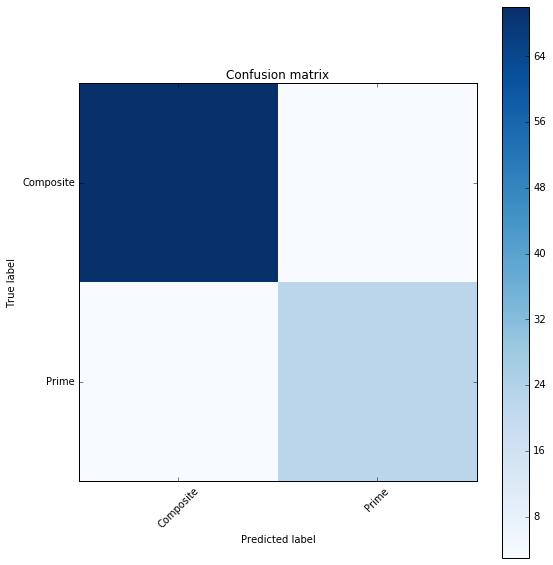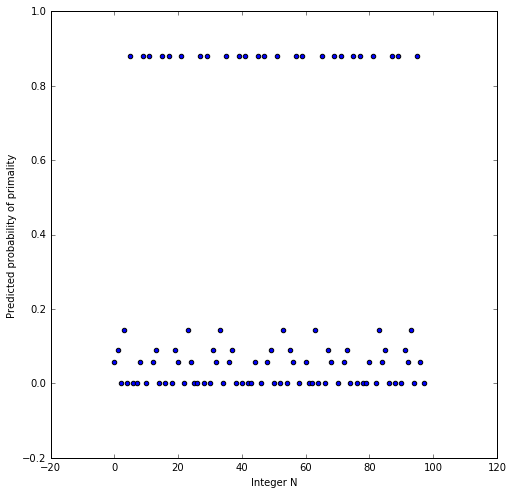Let's look at the tree.

In :
from sklearn.tree import export_graphviz
from io import StringIO
import graphviz

sio = StringIO()
export_graphviz(clf.tree_, out_file=sio,
proportion=True,
rounded=True)
graphviz.Source(sio.getvalue())

Out:

The tree is checking if the number is divisible by 2, then by 3, and finally by 5, so it's basically a prime number sieve. As the depth increases more primes are considered, so the accuracy improves.

In :
X, y = generate_data(1000)
data = []
for depth in range(2, 25):
clf = tree.DecisionTreeClassifier(max_depth=depth)
acc, cm, clf = fit_and_predict(X, y, clf)
data.append([depth, acc])

print("Final Misses:", misclassified_values(X, y, clf))
print("Final Confusion Matrix:", '\n', cm)

cdf = pd.DataFrame(data, columns=['Tree Depth', 'Accuracy'])
cdf.plot(x="Tree Depth", y="Accuracy")
# plt.plot(cdf['depth'], cdf['accuracy'])
plt.show()

Final Misses: [2, 3, 5, 7, 11]
Final Confusion Matrix:
[[830   0]
[  5 163]]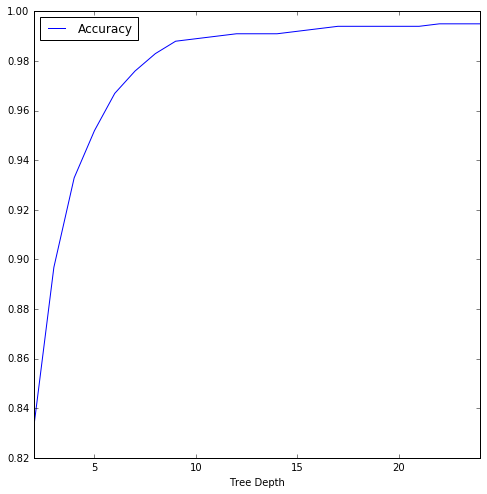If we allow the tree to be unbounded (len(primes) is deep enough), the prediction is perfect.

In :
clf = tree.DecisionTreeClassifier(max_depth=len(primes))

acc, cm, clf = fit_and_predict(X, y, clf)
print("Accuracy:", acc)
print("Misses:", misclassified_values(X, y, clf))
print("Confusion Matrix:", '\n', cm)

plot_confusion_matrix(cm)
plt.show()
plot_probabilities(X, clf)
plt.show()

Accuracy: 1.0
Misses: []
Confusion Matrix:
[[830   0]
[  0 168]]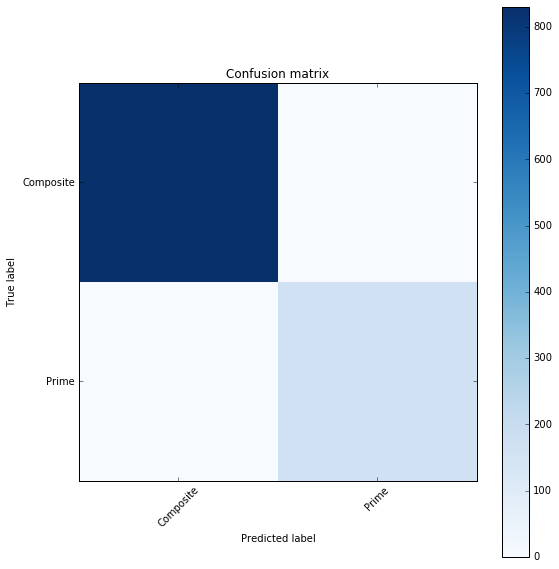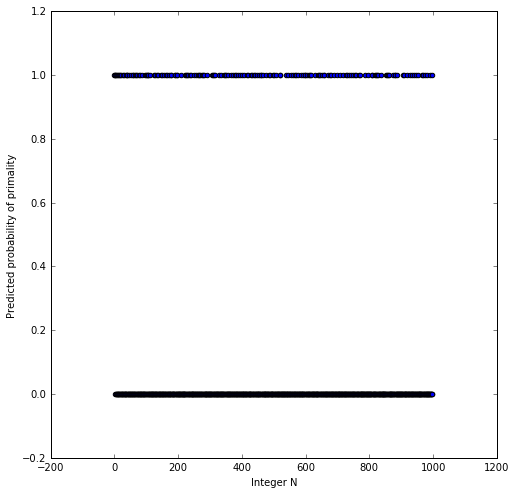### Logistic Regression¶

If we were using a linear regression, all we need to do is assign a constant parameter to each column. Any prime will only have one non-zero column with value 1, and all other columns should have a sum greater than one. In other words, we should essentially have the sum of the exponents in the prime decomposition. With a proper threshold between 1 and 2 we would have a perfect classifier.

A logistic regression is a linear regression, but on the probability of being in one class versus the other. Let's see what that looks like.

In :
from sklearn.linear_model import LogisticRegression

X, y = generate_data(400)

clf = LogisticRegression(multi_class='multinomial', solver='lbfgs',
C=1.5)

acc, cm, clf = fit_and_predict(X, y, clf)
print("Accuracy:", acc)
print("Misses:", misclassified_values(X, y, clf))
print("Confusion Matrix:", '\n', cm)

plot_confusion_matrix(cm)
plt.show()
plot_probabilities(X, clf)
plt.show()

Accuracy: 0.989949748744
Misses: [2, 3, 5, 7]
Confusion Matrix:
[[320   0]
[  4  74]]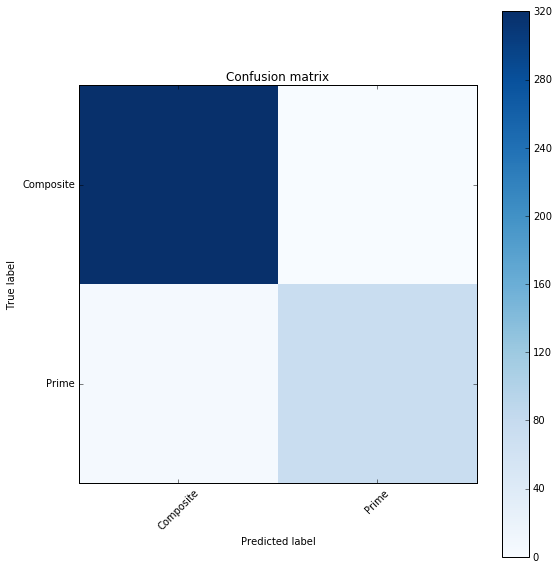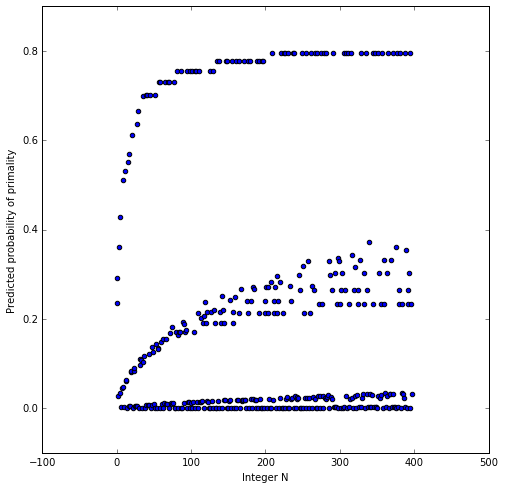As the dataset gets larger, our accuracy increases, and only the early primes are misclassified. We could combine with support vector machine (or just a linear separator) to find a diagonal line that perfectly classifies the data.

In :
X, y = generate_data(2000)

clf = LogisticRegression()
acc, cm, clf = fit_and_predict(X, y, clf)
print("Accuracy:", acc)
print("Misses:", misclassified_values(X, y, clf))
print("Confusion Matrix:", '\n', cm)

plot_probabilities(X, clf)
plt.show()

Accuracy: 0.994994994995
Misses: [2, 3, 5, 7, 11, 13, 17, 19, 23, 29]
Confusion Matrix:
[[1695    0]
[  10  293]]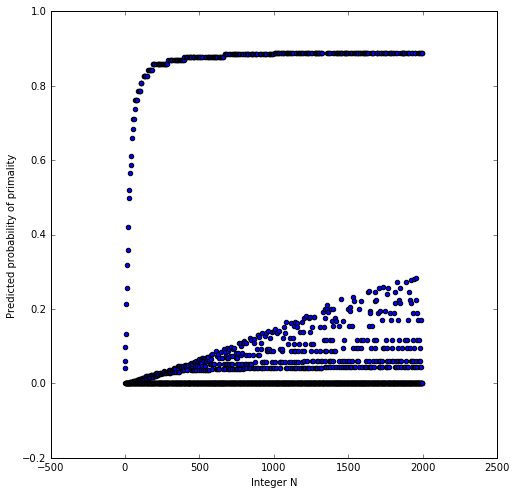### Support Vector Machine¶

A SVM also performs quite well. Since the primes lie on the corner points of the unit hyper cube, a set of bounding hyperplanes should essentially separate all the primes.

In :
from sklearn import svm
from sklearn.metrics import accuracy_score, confusion_matrix

X, y = generate_data(400)

clf = svm.SVC(kernel='linear', probability=True, C=0.5)

acc, cm, clf = fit_and_predict(X, y, clf)
print("Accuracy:", acc)
print("Misses:", misclassified_values(X, y, clf))
print("Confusion Matrix:", '\n', cm)

plot_confusion_matrix(cm)
plt.show()
plot_probabilities(X, clf)
plt.show()

Accuracy: 0.989949748744
Misses: [2, 3, 5, 7]
Confusion Matrix:
[[320   0]
[  4  74]]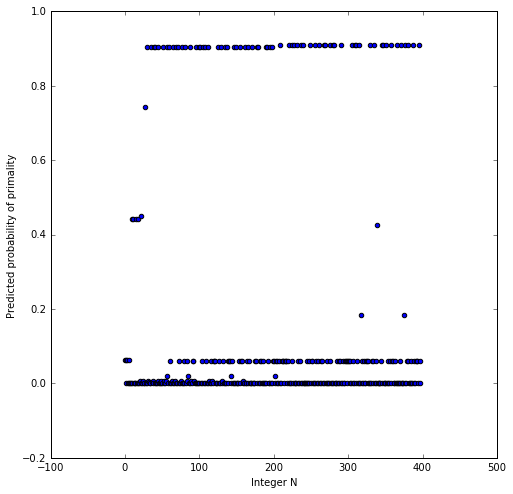## K-Neighest-Neighbors¶

KNN should work well because the prime numbers occupy the corners of the unit hypercube in our vector space. Most numbers will be relatively far away from the unit hypercube, so for a large enough $k$ (number of neighbors) we should get a very good fit.

Using distance-based weighting actually gives a perfect fit!

In :
from sklearn.neighbors import KNeighborsClassifier

X, y = generate_data(1000)

clf = KNeighborsClassifier(n_neighbors=5, weights='distance')

acc, cm, clf = fit_and_predict(X, y, clf)
print("Accuracy:", acc)
print("Misses:", misclassified_values(X, y, clf))
print("Confusion Matrix:", '\n', cm)

plot_confusion_matrix(cm)
plt.show()
plot_probabilities(X, clf)
plt.show()

Accuracy: 1.0
Misses: []
Confusion Matrix:
[[830   0]
[  0 168]]If we don't use distance-based weighting we still obtain a good fit that gets better with the neighborhood size.

In :
X, y = generate_data(1000)
data = []

for n_neighbors in range(5, 30):
clf = KNeighborsClassifier(n_neighbors=n_neighbors)

clf = clf.fit(X, y)
acc, cm, clf = fit_and_predict(X, y, clf)
data.append([n_neighbors, acc])

print("Final Misses:", misclassified_values(X, y, clf))
print("Final Confusion Matrix:", '\n', cm)

cdf = pd.DataFrame(data, columns=["n_neighbors", 'Accuracy'])
cdf.plot(x="n_neighbors", y="Accuracy")
plt.show()

Final Misses: [2, 3, 5, 7, 11, 13, 17, 19]
Final Confusion Matrix:
[[830   0]
[  8 160]]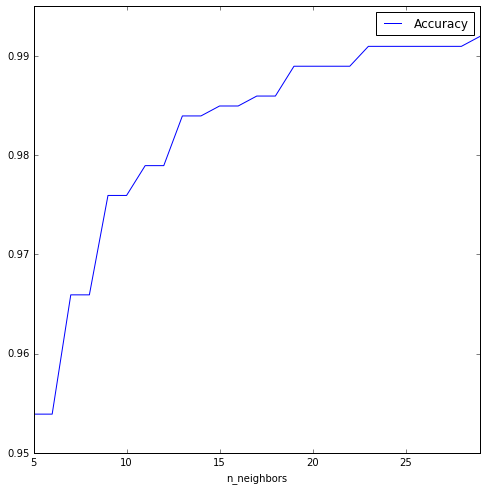## Neural Network Classifier¶

Next we use a relatively simple feed-forward neural network. This works quite well, which is no surprise given that logistic regression worked so well.

In :
from keras.models import Sequential
from keras.layers.core import Dense, Activation
from keras.optimizers import SGD   # Stochastic Gradient Descent
from keras.utils import np_utils

Using Theano backend.

In :
X, y = generate_data(10000)

y_cat = pd.get_dummies(y).values

X = np.array(X)
y_cat = np.array(y_cat)

print(X.shape, y_cat.shape)

(9998, 1229) (9998, 2)

In :
print('Compiling the model ...')
model = Sequential()

model.compile(loss='mse', optimizer=SGD(lr=0.01))

Compiling the model ...

In :
print('Training...')
loss = model.fit(X, y_cat, nb_epoch=5000, verbose=False)

loss.history['loss'][-1]   # displays MSE at last iteration
print('Training Complete')

Training...
Training Complete

In :
# Make our predictions
predictions = model.predict_classes(X, verbose=False)  # for classifiers!
print('Accuracy:', accuracy_score(y, predictions))
cm = confusion_matrix(y, predictions)
print("Confusion Matrix:", '\n', cm)

plot_confusion_matrix(cm)
plt.show()
plot_probabilities(X, model)

Accuracy: 0.999199839968
Confusion Matrix:
[[8769    0]
[   8 1221]]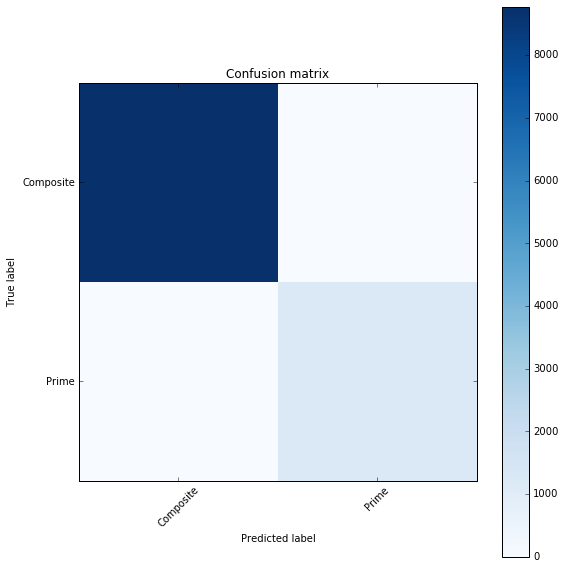9408/9998 [===========================>..] - ETA: 0s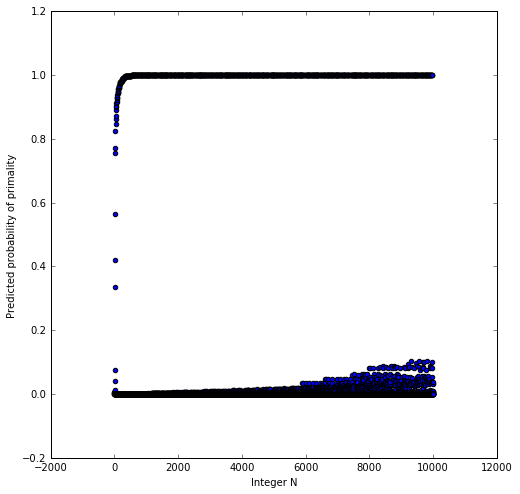In [ ]: